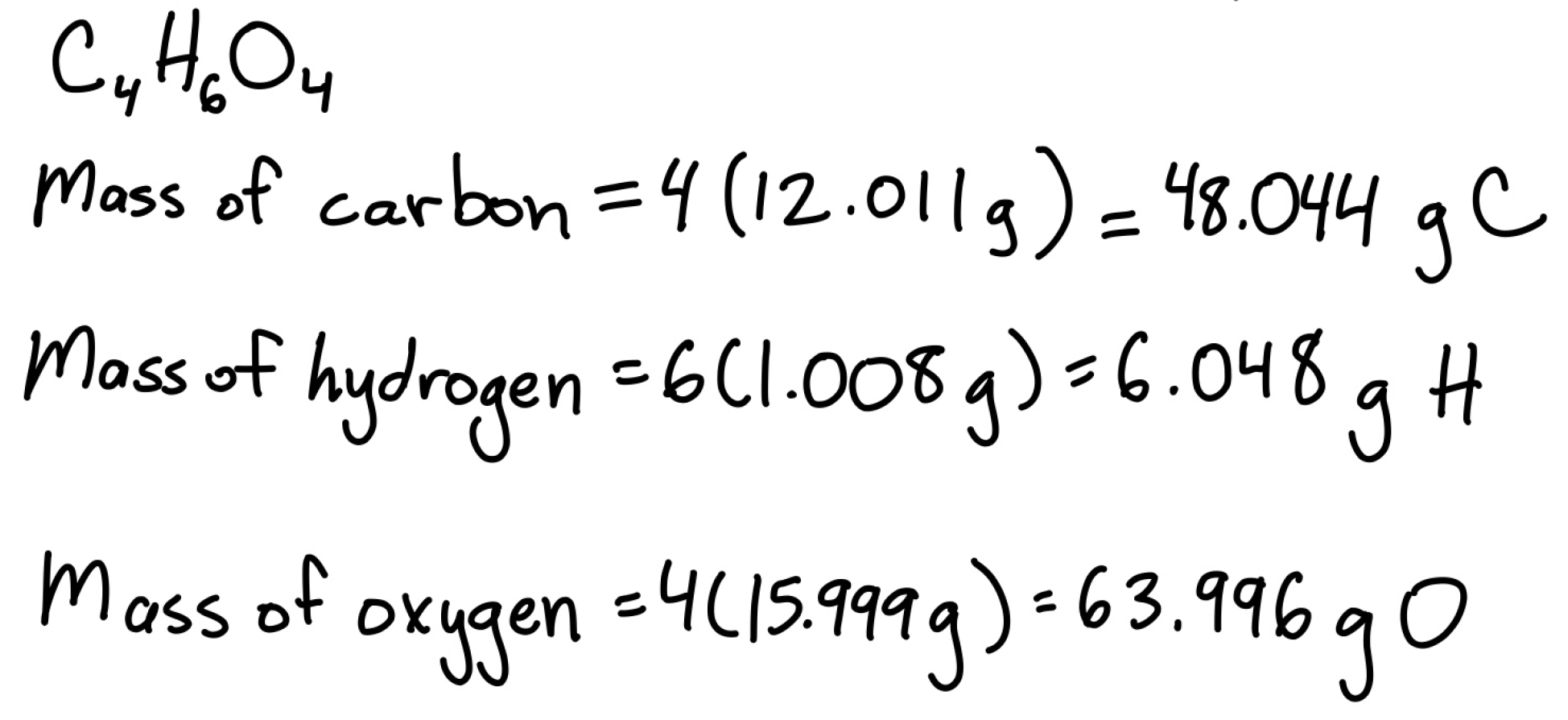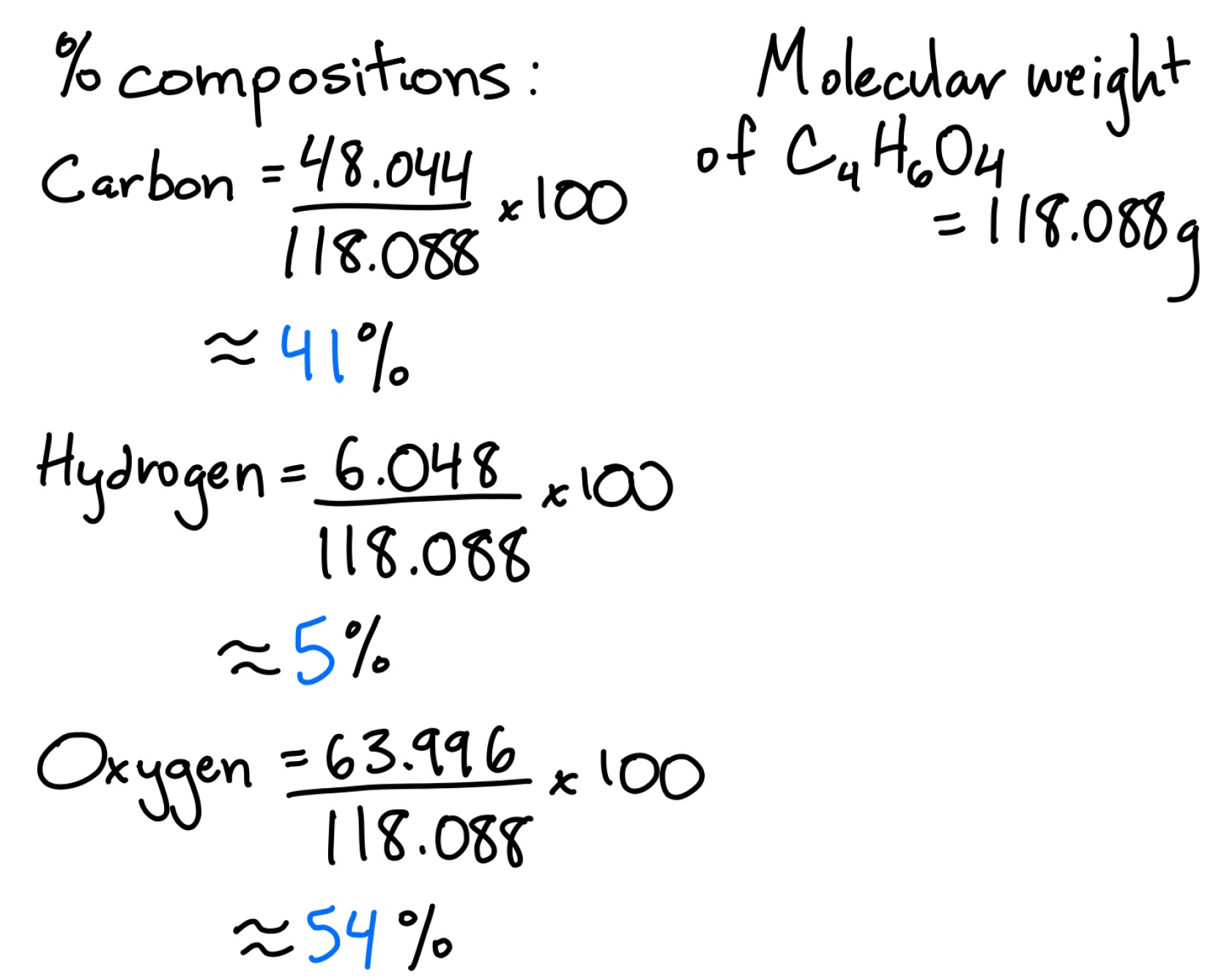Having trouble writing your personal statement?

MCAT Content / Stoichiometry / Percent Composition Of Compounds

### Description of composition by percent mass

Topic: Stoichiometry

The percent composition of compounds is the percent by mass of each element in a given compound.

The percent composition can be calculated given the molecular formula and molecular weight of a substance. The mass of the element in the compound is divided by the total mass of the compound and multiplied by 100, as seen below:For example, if we were given the molecular formula and molecular weight for succinic acid, we could find the percent composition by first determining the total mass of each element in the compound.Note that each element is multiplied by its subscript to give the total mass of the element in the compound. We can now use these values and divide each of them by the total mass and multiply by 100 to give the percent composition of each element in the compound.Note that the percent compositions should add up to 100%. In this case, 41% + 5% + 54% = 100%.

Practice Questions

Hemiacetal formation of carbohydrates

MCAT Official Prep (AAMC)

Practice Exam 2 C/P Section Passage 8 Question 41

Key Points

• The percent composition of a compound can be measured experimentally, and these values can be used to determine the empirical formula of a compound.

• The percent composition is obtained by dividing the mass of the element by the total mass of the compound and multiplying the number by 100.

• The percents of all elements in a given compound should add up to 100%.

Key Terms

molecular formula: exact number of atoms in a compound.

molecular weight: the total mass of molecules in an atom.

subscript:  number written below and immediately after an element that indicates the number of atoms of that element present.

Billing Information